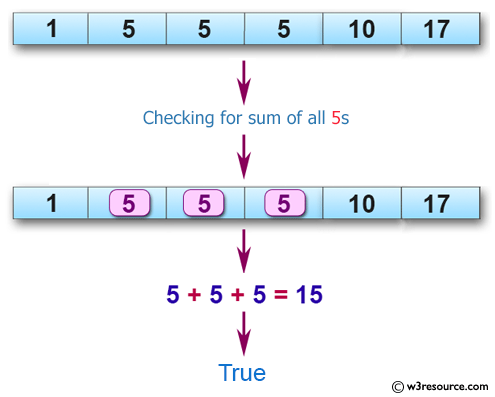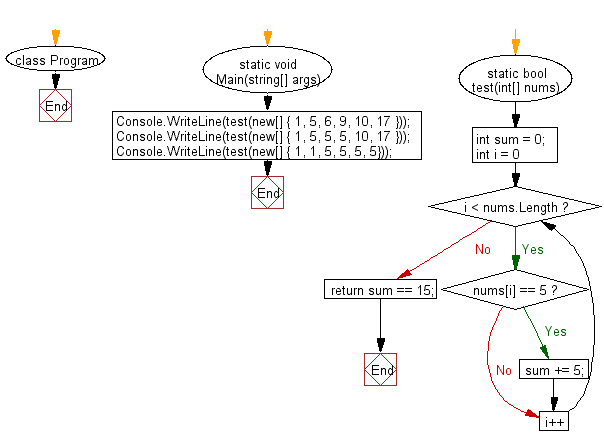﻿ C# - Check if the sum of all 5 in an array is exactly 15# C# Sharp Basic Algorithm Exercises: Check whether the sum of all 5' in the array exactly 15 in a given array of integers

## C# Sharp Basic Algorithm: Exercise-115 with Solution

Write a C# Sharp program to check whether the sum of all 5' in the array exactly 15 in a given array of integers.

Pictorial Presentation:Sample Solution:

C# Sharp Code:

``````using System;
namespace exercises
{
class Program
{
static void Main(string[] args)
{

Console.WriteLine(test(new[] { 1, 5, 6, 9, 10, 17 }));
Console.WriteLine(test(new[] { 1, 5, 5, 5, 10, 17 }));
Console.WriteLine(test(new[] { 1, 1, 5, 5, 5, 5}));

}

static bool test(int[] nums)
{
int sum = 0;

for (int i = 0; i < nums.Length; i++)
{
if (nums[i] == 5)
sum += 5;
}

return sum == 15;
}
}
}
```
```

Sample Output:

```False
True
False
```

Flowchart:C# Sharp Code Editor:

Improve this sample solution and post your code through Disqus

What is the difficulty level of this exercise?

Test your Programming skills with w3resource's quiz.

﻿